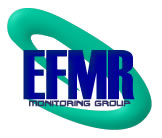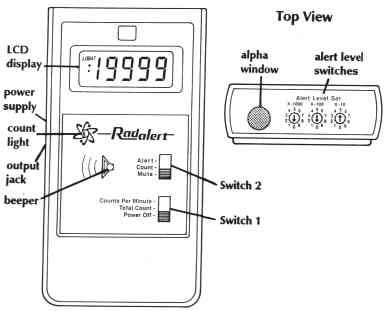Home | RadAlert Monitor RadAlert Monitor RadAlert Diagram The RadAlert can count up to 19,999 ionizing events per minute.A red diode flashes on each count. A speaker can be activated to click on each count just like the classic geiger counter. An alarm can be activated to alert the user if radiation levels reach a preset amount selected by the user. A count of 1000 is approximately equal to 1 millirad per hour. The RadAlert detects Alpha, Beta, Gamma and X-Ray radiation. Sometimes the red diode will flash three or four times in very rapid succession as it detects a cosmic ray. This is called a cosmic shower and is a source of gamma radiation from space. Radbasics: Units of Measurement At first, the units and methods for measuring radiation can seem confusing. If you keep in mind a few simple rules, they are easily demystified. The first thing to remember is that just like other ways we can measure things, there are two different standards and sets of units used for the same type of measurement [an international system unit (SI), and a U.S.- British unit]. The table below separates these two appropriately. Measurement International (SI) Unit U.S. Unit Conversions Radioactivity Becquerel (Bq) Curie (Ci) 3.7 x 1010 Bq = 1 Ci) Absorbed Dose Gray (Gy) RAD 1 Gy = 100 RADs Equivalent Dose Sievert (Sv) REM 1 Sv = 100 REMs The second thing to remember is that the main units for radiation dosage fall under 2 definitions. "Absorbed dose" is simply a measurement of how much radiation gets absorbed by something. "Equivalent dose" takes the amount that gets absorbed by something, and factors in the intensity of the radiation to give us an idea of how much damage has been done. Absorbed Dose: RADs and Grays This relates to the amount of energy actually absorbed in some material, and is used for any type of radiation and any material. Rads describe doses from both external penetrating radiation and from radionuclides contained within the body, but do not measure specific biological damage. A rad to the hand, for example, is not considered as dangerous as a rad distributed over the whole body. A RAD is the american unit used to measure absorbed dose, and stands for "Radiation Absorbed Dose". One RAD is defined as the absorption of 100 ergs per gram of material, and amounts to approximately one ionization per cubic micron. A gray is the SI unit used to measure absorbed dose. One gray is equal to one joule of energy deposited in one kg of a material. Absorbed dose is often expressed in terms of hundredths of a gray, or centi-grays. One gray is equivalent to 100 RAD. Equivalent Dose : REMs and Sieverts Equivalent dose is considered the most appropriate means for measuring biological damage from radiation. It reflects the fact that some forms of radiation create more damage in a given exposure than others; that not all radiation has the same biological effect, even for the same amount of previously mentioned absorbed dose. (for example, one gray of alpha radiation will have a greater effect than one gray of beta radiation) This measurement is calculated by multiplying the absorbed dose by modifying factors calculated on considerations of type of ionizing radiation and radiosensitivity of the tissues involved. This is measured by REMs in the United States, and by Sieverts in the international community. A REM is the american unit used to measure equivalent dose, and stands for "Roentgen Equivalent Man" ("man" was later adjusted for political correctness to "mammal"). Equivalent dose using rems is often expressed in terms of thousandths of a rem, or millirem. To determine equivalent dose (rem), you multiply absorbed dose (RAD) by a quality factor (Q) that is unique to the of incident radiation. With Sieverts, equivalent dose is often expressed in terms of millionths of a Sievert, or micro-Sievert. Just as with the american REM, to determine equivalent dose (Sv), you multiply absorbed dose (Gy) by a quality factor (Q) that is unique to the of incident radiation. One Sievert is equivalent to 100 REM. Again, these measurements relate the absorbed dose in human tissue to the effective biological damage of the radiation. When radiation doses are measured for large populations, the unit person rem is used. This is calculated by multiplying the total number of people exposed times their average dose in rems. Or it can be the actual sum of all the doses they receive. For example, ten thousand person rems is a dose received by five thousand people exposed to two rems each; or by ten thousand people exposed to one rem each. According to Dr. John Gofman, at least one death will result for every 300 person-rem dose. The nuclear industry has said some 2000 person rems escaped at Three Mile Island. In terms of measuring X and gamma rays, the rad and the rem are the same relative to their biological damage potential. But alpha- and beta-emitters do much more biological damage when they are taken inside the body and lodged in sensitive tissues than when there is exposure just from outside the body. The equivalent dose rem measurement factors in these differences. Measuring Radioactivity Itself: Curies and Becquerels It is necessary to measure gross radioactivity in a substance which does not relate to doses or biological damage. The complimentary units of measurement for accomplishing this are the american Curie (Ci), and the international Becquerel (Bq). These both measure the rate at which radioactive material decays or transforms per second. It is during this process of decay that radioactive material emits the different types of radiation we speak of, and thus these units provide a yardstick for measuring the radiation emitted. [for more information on the process of decay, please refer to the section on radioactive decay and half-life]. One curie is the amount of radioactivity in one gram of the element first discovered by Madame Curie, Radium. It is also the quantity of a radioactive material that will have 37,000,000,000 disintegrations of decay in one second. Often, measurements using curies are expressed in smaller units like: thousandths (mCi), one millionths (µCi) or even billionths (nCi) of a curie. There are many billion curies of radioactivity in an atomic reactor. One curie is the equivalent of 3.7 x 1010 becquerels. One Becquerel is that quantity of a radioactive material that will have 1 transformation in one second. Often, measurements using Becquerels are expressed in larger units like: thousands (kBq), millions (MBq) or even billions (GBq) of a Becquerel. As a result of having one Becquerel being equal to one transformation per second, there are 3.7 x 1010 Bq in one curie. The Roentgen /rent' gen/ Lastly, the Roentgen(R) is a unit used to measure a quantity called exposure. Not to be confused with dose, this can only be used to describe an amount of gamma and X-rays, and only in the air. One Roentgen is equal depositing to 2.58 x 10-4 coulombs per kg of dry air. It is a measure of the ionizations of the molecules in a mass of air. The main advantage of this unit is that it is easy to measure directly, but it is limited because it is only for deposition in air, and primarily effective for gamma and x-rays. Roentgens are often seen as interchangable with RADs and REMs when it comes to gamma rays and x-rays. It also works out that for a "rough" estimate for beta, x-ray, and gamma sources, 1 R = 1 rad = 1 rem = 10 mSv. The following tables will aid in working with these units. The first table shows the different prefixes used with units of measurement. The second table below it lists some further conversions for the units of measurement we just learned about, to help us quickly compare values as we read further on the subject of ionizing radiation and the relations of radioactive materials. Prefixes Used With Units of Measurement yotta [Y] 1 000 000 000 000 000 000 000 000 = 10^24 zetta [Z] 1 000 000 000 000 000 000 000 = 10^21 exa [E] 1 000 000 000 000 000 000 = 10^18 peta [P] 1 000 000 000 000 000 = 10^15 tera [T] 1 000 000 000 000 = 10^12 giga [G] 1 000 000 000 (a thousand millions = a billion) mega [M] 1 000 000 (a million) kilo [k] 1 000 (a thousand) hecto [h] 100 (a hundred) deca [da] 10 (ten) - - 1 - deci [d] 0.1 (a tenth) centi [c] 0.01 (a hundredth) milli [m] 0.001 (a thousandth) micro [µ] 0.000 001 (a millionth) nano [n] 0.000 000 001 (a thousand millionth) pico [p] 0.000 000 000 001 = 10^-12 femto [f] 0.000 000 000 000 001 = 10^-15 atto [a] 0.000 000 000 000 000 001 = 10^-18 zepto [z] 0.000 000 000 000 000 000 001 = 10^-21 yocto [y] 0.000 000 000 000 000 000 000 001 = 10^-24 Useful Radiation Conversions 1 terabecquerel (TBq) ~ 27 curie (Ci) 1 gigabecquerel (GBq) ~ 27 millicurie (mCi) 1 megabecquerel (MBq) ~ 27 microcurie (µCi) 1 kilobecquerel (kBq) ~ 27 nanocurie (nCi) 1 becquerel (Bq) ~ 27 picocurie (pCi) = 1 dps 1 curie (Ci) ~ 37 gigabecquerel (GBq) 1 millicurie (mCi) ~ 37 megabecquerel (MBq) 1 microcurie ~ 37 kilobecquerel (kBq) 1 nanocurie (nCi) ~ 37 becquerel (Bq) 1 picocurie (pCi) ~ 37 millibecquerel (mBq) 1 Gray (Gy) = 100 rad (rad) 1 milligray (mGy) = 100 millirad (mrad) 1 microgray (µGy) = 100 microrad (µrad) 1 nanogray (nGy) = 100 nanorad (nrad) 1 kilorad (krad) = 10 gray (Gy) 1 rad (rad) = 10 milligray (mGy) 1 millirad (mrad) = 10 microgray (µGy) 1 microrad (µrad) = 10 nanogray (nGy) 1 coulomb/kg (C/kg) ~ 3876 roentgen (R) 1 millicoulomb/kg (mC/kg) ~ 3876 milliroentgen (mR) 1 microcoulomb/kg (µC/kg) ~ 3876 microroentgen (µR) 1 nanocoulomb/kg (nC/kg) ~ 3876 nanoroentgen (nR) 1 kiloroentgen (kR) ~ 258 millicoulomb/kg (mC/kg) 1 roentgen (R) ~ 258 microcoulomb/kg (µC/kg) 1 milliroentgen (mR) ~ 258 nanocoulomb/kg (nC/kg) 1 microroentgen (µR) ~ 258 picocoulomb/kg (pC/kg) 1 sievert (Sv) = 100 rem (rem) 1 millisievert (mSv) = 100 millirem (mrem) 1 microsievert (µSv) = 100 microrem (µrem) 1 kilorem (krem) = 10 sievert (Sv) 1 rem (rem) = 10 millisievert (mSv) 1 millirem (mrem) = 10 microsievert (µSv) Other Helpful Conversion Factors I was previously going to post a table here with conversion factors, but soon found that the table would be annoyingly huge, and I wanted it to be as complete as possible. What I have decided to do instead is to refer my readers to a section of the National Institute of Standards and Technology's (NIST) website, The Reference on Constants, Units, and Uncertainty. They have compiled a huge listing of conversions for just about every concievable unit of measurement out there, and additionally make it available in a very nicely formatted PDF. Below is the diagram of the RadAlert:© 2003-2008 EFMR Monitoring Group